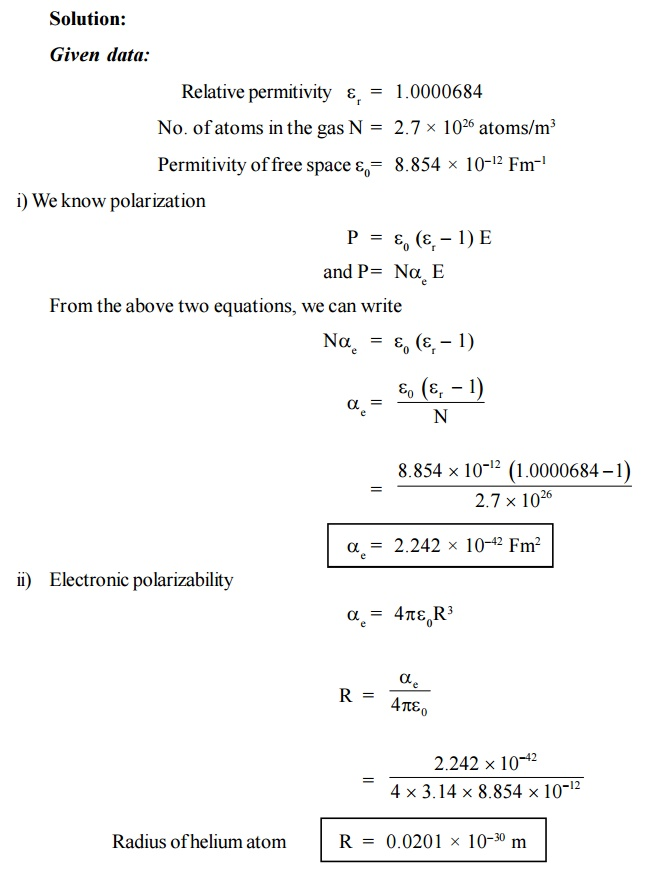Home | | Engineering Physics II | Solved Problems: Dielectric Materials

# Solved Problems: Dielectric Materials

Physics - Dielectric Materials - Important Solved Problems(Sum): Dielectric Materials

SOLVED PROBLEMS

1.Find the capacitance of a layer of Al2O3that is 0.5 m thick and 2000 mm2 of square area ( r = 8.854 × 10–12 Fm–1)

Solution:

Given data:2.A layer of porcelain is 80 mm long, 20 mm wide and 0.7 µm thick. Calculate its capacitance with r = 6

Solution:

Given data:3.If an ionic crystal is subjected to an electric field of 1000 Vm–1 and the resulting polarization 4.3 × 10–8 cm2. Calculate the relative permittivity of NaCl. Solution:

Given data:4.Calculate the electronic Polarization of argon atom. Given at r = 1.0024 at NTP and N = 2.7 × 1025 atom m–3 .

Solution :

Relative permitivity = 1.0024 at NTP

Permitivity of free space  = 8.854 × 10–12 Fm–15.A solid contains 5 × 1028 atoms/m3 each with a polarisability of 2×10–40 F m2. Assuming that the internal field is given by Lorentz formula. Calculate the ratio of internal field to the external field. 0 = 8.854 × 10–12 Fm–1.6.  Calculate the polarization produced in dielectric medium of dielectric constant 6 when it is subjected to an electric field of 100 Vm–1. (e0  = 8.854 × 10–12 Fm–1)P = 4.425 × 10–9 Vm–2 F

7. Calculate the electronic polarizability of neon. The radius of neon atom is 0.158 nm. (  = 8.854 × 10–12 Fm–1)8.The dielectric constant of a helium gas at NTP is 1.0000684. Calculate the electron polarizability of helium atoms if the gas contains 2.7 × 1026 atoms/m3 and hence calculate the radius of helium atom ( = 8.854 × 10–12 Fm–1)

Solution:1.  The electronic polarisability is 0.18x 10 40 fm2. Find the relative dielectric constant at 0C and 1 atmospheric pressure.   (Ans: 1.000055)

A capacitor has capacitance of 0.019 F when uses wax paper r 1.85 between the electrodes of Aluminum foil. The wax paper is to be replaced by plastic film r 2.15 of same dimensions. Taking other factors being equal, obtain the change (increase/decrease) in capacitance.

(Ans: Increase of 0.0117 F)

A capacitor uses Aluminum oxide as the dielectric with relative permeability = 8. An effective surface area of 360 cm2 gives a capacitance of 6 F . Calculate the field strength and the total dipole moment induced in oxide layer if a potential difference of 15 volts exist across the capacitor.

(Ans: (i) 3.535 × 107 V/m (ii) 33.4 x 10 C – m)

The radium of the Helium atom is about 0..55 AU. Calculate the polarisability of Helium and its relative permeability. The number of Helium atoms in a volume of 1 m is 2.7 × 1025 atoms. (Ans: (i) 0.185 × 10–40 Farad – m² (ii) 1.000057)

Study Material, Lecturing Notes, Assignment, Reference, Wiki description explanation, brief detail
Physics : Dielectric Materials : Solved Problems: Dielectric Materials |